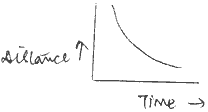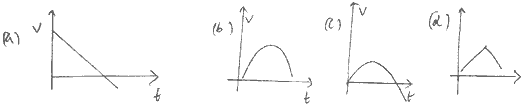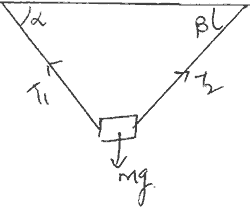Thursday , September 24 2020# 9th CBSE Science Examination: Mid Term 2019

## 9th CBSE Science Examination: Mid Term Question Paper (2019-20)

School Name: Himalaya Public School, Sector 13, Rohini, Delhi 110085 India
Time: 3 Hrs.
Marks: 70
Class: 9th
Date: 17/9/2019
Subject: Science

## General Instructions:

1. All questions are compulsory. There are 37 questions in all.
2. This question paper has four sections. Section A, Section B, Section C and Section D.
3. Section A contains 20 questions of one mark each, Section B contains 7 questions of marks each, Section C contains 7 questions of 3 marks each and Section D contains 3 questions of 5 marks each.
4. There is no overall choice. However an internal choices have been provided in 1 question of 2 marks, 2 questions of 3 marks each and all the questions of 5 marks. You have to attempt only one of the choices in such questions.

## Section A

#### Question: 5. What does the following graph signify? Is it possible or not?1. 1.5 m/s
2. 60 m/s
3. 0.1 m/s
4. 5 m/s

#### Question: 18. Which one of the following pairs of quantities has the same dimension?

1. Force and work done
2. Momentum and impulse
3. Pressure and force
4. Surface tension and stress

#### Question: 19. A particle is thrown above, then correct vt graph will be## Section C

#### Question: 31. A body of mass m is suspended by two strings making angles α and β with the horizontal as shown in fig. Find the tensions in the string.## Section D

#### Question: 37. In successive measurements, the readings of the period of oscillation of a simple pendulum were found to be 2.635, 2.565, 2.425, 2.715, 2.805 sec in an experiment.

Calculate:

1. Mean value of the period of oscillation absolute error in each measurement
2. Mean absolute error, relative error, percentage error.

## Light: 7th Class NCERT CBSE Science Chapter 15

Light 7th Class NCERT CBSE Science Chapter 15 Question: Briefly mention whether mirror change the direction of …